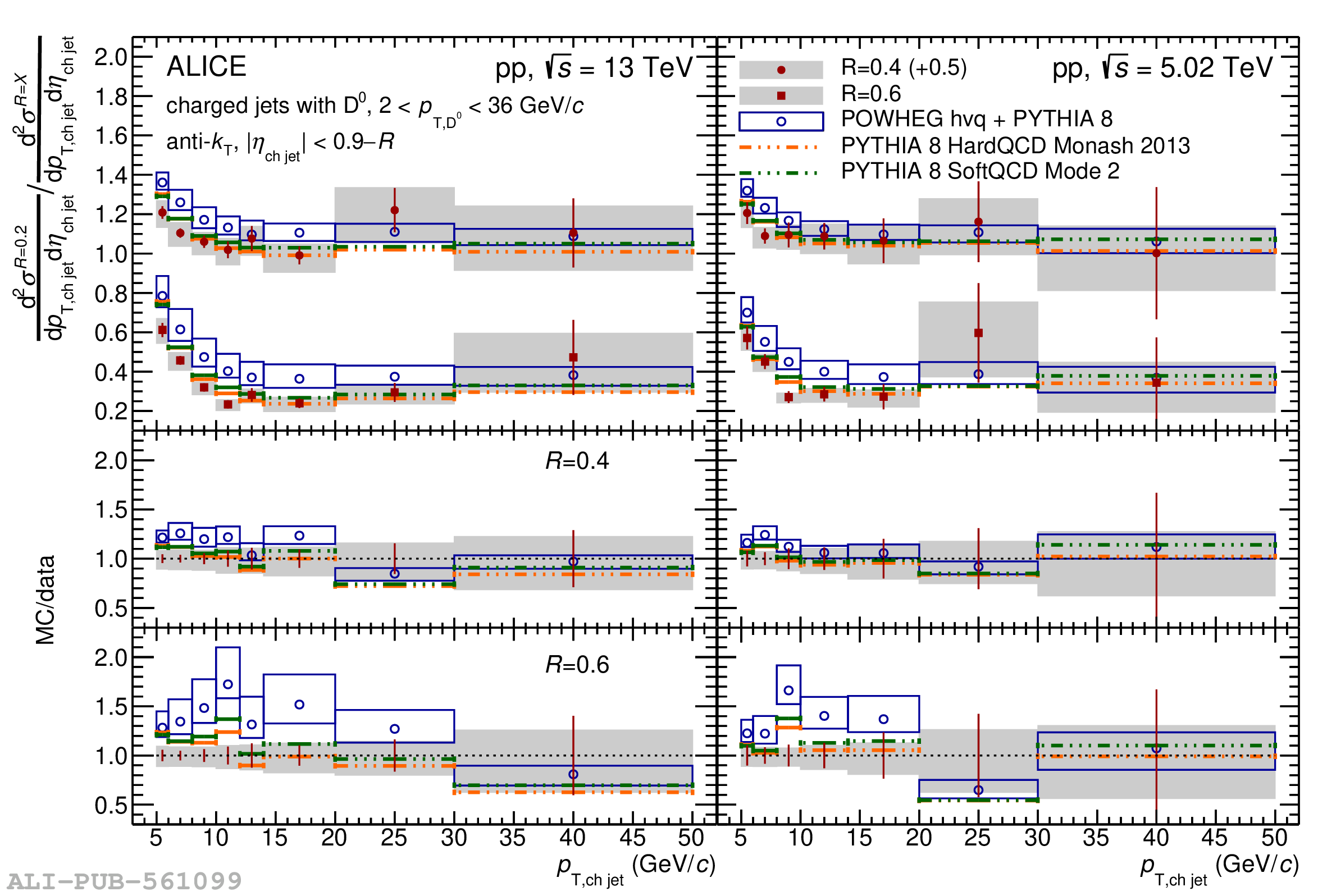# Figure 9

 Top: ratios of $p_{\mathrm{T,ch\,jet}}$-differential cross section of charm jets tagged with $\mathrm{D^{0}}$ mesons for different $R$: $\sigma(R=0.2)/\sigma(R=0.4)$ (circles, shifted up by 0.5) and $\sigma(R=0.2)/\sigma(R=0.6)$ (squares) in pp collisions at $\sqrt{s}$ = 13 TeV (left) and $\sqrt{s}$ = 5.02 TeV (right) compared to PYTHIA 8 HardQCD Monash 2013 (dash-dotted lines), PYTHIA 8 SoftQCD Mode 2 (dashed lines), and POWHEG hvq + PYTHIA 8 (open circles) predictions. The shaded bands indicate the systematic uncertainty on the cross section ratios while open boxes represent the theoretical uncertainties on the POWHEG predictions. Bottom: ratios of MC predictions to the data for $\sigma(R=0.2)/\sigma(R=0.4)$ and $\sigma(R=0.2)/\sigma(R=0.6)$, respectively.